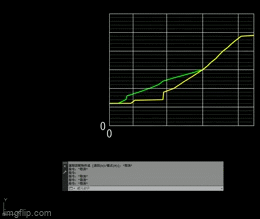Jump to content# Edit LISP ( how to change Increment way)

## Recommended PostsPosted (edited)

Dear ALL:

This LISP "DCDD" , it is according to Y axis (vertical) to increment the number.  and with TEXT type (0K+00   0K+01  0K+01.55  ......)

```;; free lisp from cadviet.com
;;; this lisp was downloaded from http://www.cadviet.com/forum/index.php?showtopic=56086&pid=171947&st=0&#entry171947
;;;;;;  https://www.youtube.com/watch?v=3-i4i1p3cwM

(defun c:dcdd (/ lstSS txtstr p1 p2 listname txt txt1 ss)
(vl-load-com)

(defun acet-ss-to-list (ss / n e l)
(setq n (sslength ss))
(while (setq e (ssname ss (setq n (1- n))))
(setq l (cons e l))
)
)

;Gan gia tri goc
(if (not k0) (setq k0 1));;gan gia tri goc
(setq k (getreal (strcat "\nDrawing Ratio:1/" (rtos k0 2 0) "")));Nhap ty le ban ve
(if (not k) (setq k k0) (setq k0 k))
(defun dowith(lstSS / lstSS en str)
(cond  ((setq en  (car (vl-remove-if-not '(lambda(x)(wcmatch (cdadr (entget x))"*TEXT")) lstSS)))(setq str (acet-dxf 1 (entget en)) en (vlax-ename->vla-object en)))
((setq en (car (vl-remove-if-not '(lambda(x)(and (wcmatch (cdadr (entget x))"INSERT")(= (acet-dxf 66 (entget x)) 1))) lstSS)))
(setq str (vla-get-textstring (setq en(car (vlax-invoke (vlax-ename->vla-object en) 'GetAttributes)))))
)
)
(cons en str)
)
(grtext -1 "Edit By Nguy\U+1EC5n Ng\U+1ECDc S\U+01A1n")
(setq  lstSS (acet-ss-to-list (setq ss (ssget)))
obj (car (setq en (dowith lstSS)))
str (cdr en)
p1 (getpoint "\nBase Point:")
eL (entlast)
oDz (getvar "Dimzin")
)
(setvar "DIMZIN" 0)
(while (setq p2 (getpoint p1 "\nNext Point:"))
(command "copy" ss "" p1 p2)
(while (setq EL (entnext EL)) (setq Listname (cons EL Listname)))
(setq  Txt1 (car (dowith listName))
eL (entlast)
)
(Ktra)
(setvar "cecolor" "bylayer")
(vla-put-textstring txt1
(strcat (cond ((> (setq num (+ (atof str) (/ (- (cadr p2)(cadr p1)) k))) 0) "0K+")
((= num 0) "%%p")
(T "")
)
(rtos num  2 2));So chu so dau dau ;
)
)
(setvar "DIMZIN" oDZ)
)
;Tim va tao moi Layer
(defun ktra ()
(if (not (tblsearch "layer" "Caodo"))
(command "-LAYER" "m" "Caodo" "c" 1 "Caodo" "" )
(setvar "clayer" "Caodo" )
)
)```

But i want to change to according to X axis (horizontal) . How to edit?

Here is another LISP " CPT"  is according to distance to increment the number.

```(defun c:cpt (/ ss a b dis eg)
(setq ss (vl-remove-if 'listp (mapcar 'cadr (ssnamex (ssget '((0 . "*TEXT"))))))
a (getpoint "\nBase Point：")
)
(setvar 'cmdecho 0)
(while (setq b (getpoint a  "\nNext Point："))
(setq dis (distance a b))
(princ (strcat "" (rtos dis)))
(foreach v ss
(command "copy" v "" "non" a "non" b)
(setq  eg (entget (entlast)))
(entmod (subst (cons 1 (rtos (+ dis (atof (cdr (assoc 1 eg)))))) (assoc 1 eg) eg))
)
)
(setvar 'cmdecho 1) (princ)
)```

Unfortunately, it is no with TEXT (like 0K+  ) and  NOT second decimal place..

How To Comprehensive The two LISP

Please Helep me

```
```
Edited by edmondsforum

#### Share this post

##### Share on other sitesSimple..... for your 'DCDD'

You Change This Line 'cadr to car'

(strcat (cond ((> (setq num (+ (atof str) (/ (- (cadr p2)(cadr p1)) k))) 0) "0K+")

(strcat (cond ((> (setq num (+ (atof str) (/ (- (car p2)(car p1)) k))) 0) "0K+")

#### Share this post

##### Share on other sitesDear Kajanthan , thanks ur help

But when i choose next point , it shows " no function definition "

Further Explanation.

Here is "DCDD" operation , that horizontal is still  ZERO

So I would like to the "CPT" function could write into "DCDD"#### Share this post

##### Share on other sitesSorry , about THE GIF is wrong (above)

Here is new : https://gph.is/g/4V8bVvo

#### Share this post

##### Share on other sitesSorry @edmondsforum

I did not check your lisp.

Now, I modified some,

Now you can apply both ways with this lisp.

```(defun c:dcdd (/ lstSS txtstr p1 p2 listname txt txt1 ss)
(vl-load-com)

(defun acet-ss-to-list (ss / n e l)
(setq n (sslength ss))
(while (setq e (ssname ss (setq n (1- n))))
(setq l (cons e l))
)
)

;Gan gia tri goc
(if (not k0) (setq k0 1));;gan gia tri goc
(setq k (getreal (strcat "\nDrawing Ratio:1/" (rtos k0 2 0) "")));Nhap ty le ban ve
(if (not k) (setq k k0) (setq k0 k))
(defun dowith(lstSS / lstSS en str)
(cond  ((setq en  (car (vl-remove-if-not '(lambda(x)(wcmatch (cdadr (entget x))"*TEXT")) lstSS)))(setq str (acet-dxf 1 (entget en)) en (vlax-ename->vla-object en)))
((setq en (car (vl-remove-if-not '(lambda(x)(and (wcmatch (cdadr (entget x))"INSERT")(= (acet-dxf 66 (entget x)) 1))) lstSS)))
(setq str (vla-get-textstring (setq en(car (vlax-invoke (vlax-ename->vla-object en) 'GetAttributes)))))
)
)
(cons en str)
)
(grtext -1 "Edit By Nguy\U+1EC5n Ng\U+1ECDc S\U+01A1n")
(setq  lstSS (acet-ss-to-list (setq ss (ssget)))
obj (car (setq en (dowith lstSS)))
str (cdr en)
p1 (getpoint "\nBase Point:")
eL (entlast)
oDz (getvar "Dimzin")
)
(setvar "DIMZIN" 0)
(while (setq p2 (getpoint p1 "\nNext Point:"))
(command "copy" ss "" p1 p2)
(while (setq EL (entnext EL)) (setq Listname (cons EL Listname)))
(setq  Txt1 (car (dowith listName))
eL (entlast)
)
(Ktra)
(setvar "cecolor" "bylayer")
(vla-put-textstring txt1
(strcat (cond ((> (setq num (+ (atof str) (/ (distance p2 p1) k))) 0) "0K+")
((= num 0) "%%p")
(T "")
)
(rtos num  2 2));So chu so dau dau ;
)
)
(setvar "DIMZIN" oDZ)
)
;Tim va tao moi Layer
(defun ktra ()
(if (not (tblsearch "layer" "Caodo"))
(command "-LAYER" "m" "Caodo" "c" 1 "Caodo" "" )
(setvar "clayer" "Caodo" )
)
)```#### Share this post

##### Share on other sites@Kajanthan thanks u very much!! it's works!!

## Join the conversation

You can post now and register later. If you have an account, sign in now to post with your account.
Note: Your post will require moderator approval before it will be visible.Reply to this topic...

×   Pasted as rich text.   Paste as plain text instead

Only 75 emoji are allowed.

×   Your link has been automatically embedded.   Display as a link instead

×   Your previous content has been restored.   Clear editor

×   You cannot paste images directly. Upload or insert images from URL.

×

×
×
• Create New...